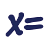Games
Problems
Go Pro!

# Counting Principles

Reference > Mathematics > AlgebraHow to determine the number of ways in which two or more events can happenHow to count events when one event affects the nextPermutations - Explanation and mathematical formula for number of arrangmentsCalculate the number of permutations if there are repeated objectsCombinations - how to count arrangements when order doesn't matterLike us on Facebook to get updates about new resources